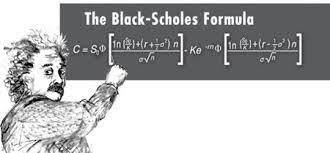Today we will be pricing a vanilla call option using a monte carlo simulation in Python. Monte Carlo models are used by quantitative analysts to determine accurate and fair prices for securities. Typically, these models are implemented in a fast low level language such as C++. However, for the sake of ease, we’ll be using Python.

## Pre-Requisites:

Below is a list of pre-requisite knowledge to get the most out of this tutorial.

### Required:

• Calculus
• Probability and Statistics
• Very basic programming
• Stochastic Processes
• Stochastic Calculus or an introductory asset pricing class

## Understanding The Math

Before diving into the code, we’ll cover some of the basic financial mathematics necessary to understand the model.

### Assumptions

In our model we will make the following assumptions.

• The price of our stock will generally increase with respect to time
• The expected return is a fixed rate of the current share price
• The stock follows a random-walk behavior

### The Stock Price Evolution Model

We will be using a stochastic differential equation as the model of our stock price evolution. It will consist of a component representing the expected return on the stock $S_{t}$ over an infintesimal period of time $dt$, represented by:

$\mu S_{t}dt$

Where $S_{t}$ is the price of our share with respect to time, and $\mu$ is our expected rate of return.

To make our model representative of real share price behavior we must also add a stochastic element represented by a fixed fraction of our price $\sigma$, our share price $S_{t}$ and a random walk process $dW_{t}$ to construct

$\sigma S_{t} dW_{t}$

Altogether, we get the equation:

$dS_{t} = \mu S_{t}dt + \sigma S_{t} dW_{t}$

### Black-Scholes Option Pricing Model

The Black-Scholes option pricing model tells us the the price of a vanilla option with a compounding rate $r$ , expiration $T$ and payoff function $f$ is: $e^{-rT}E(f(S_{T}))$

#### Solving for Expectation

In order to finish deriving an expression for our fair options price, we must solve the expression within the expected payoff function. To begin with, we will use a risk-neutral stochastic differential equation (see stock price evolution model) for the expectation of $S_{t}$:

$dS_{t} = rS_{t}dt + \sigma S_{t}dW{t}$

By taking the log of both sides of the equation and using Ito’s lemma, a solution to the stochastic process:

$d\log(S_{t}) = (r - \frac{1}{2}\sigma^2)dt + \sigma dW_{t}$

substituting a constant coefficient for d, (a convinient model simplification) we will obtain the final expression:

$d\log(S_{t}) = log(S_{0}) + (r - \frac{1}{2}\sigma^2)t + \sigma W_{t}$

$W_{t}$ is a brownian motion process with a mean $0$ and variance $T$ and normally distributed random variable $N(0,1)$:

$W_{T} = \sqrt{T}N(0,1)$

Our final expression for $S_{t}$ is:

$log(S_{T}) = log(S_{0}) + (r - \frac{1}{2}\sigma^2)T + \sigma \sqrt{T}N(0,1)$

Raising the $e$ to the equation, we can simplify to:

$S_{T} = S_{0}e^{(r - \frac{1}{2}\sigma^2)T + \sigma \sqrt{T}N(0,1)}$

We will continously sample random numbers for $N(0,1)$ into the equation while maintaining a running sum:

$f(S) = (S - K)_{+}$

Where $K$ is the strike price. The average of this expression where n is the number of random samples we take will be

$\frac{\sum_{i}^{n} (S_{0}e^{(r - \frac{1}{2}\sigma^2)T + \sigma \sqrt{T}N(0,1)} - K)}{n}$

#### Final Expression

Our final expression for the fair price of the option will be the above expression multiplied by $e^{-rT}$ to complete our original black scholes model.

$e^{(-rT)}\frac{\sum_{i}^{n} (S_{0}e^{(r - \frac{1}{2}\sigma^2)T + \sigma \sqrt{T}N(0,1)} - K)}{n}$

## The Code

import math
import random
import numpy

##Simple Monte Carlo Pricing Class for Vanilla Call Option
class SimpleMCPricer():
def __init__(self, expiry, strike, spot, vol, r, paths):
#The sigma value on the left side of the exponent
self.variance = vol**2 * expiry
#The sigma value on the right side of the e exponent
self.root_Variance = math.sqrt(self.variance)
#Corresponds to the (-1/2 * sigma^2)
self.itoCorr = -0.5*self.variance
##Corresponds to S0e^(rT - 1/2 sigma^2T)
self.movedSpot = spot*math.exp(r*expiry + self.itoCorr)
self.runningSum = 0
##Simulate for all paths
for i in range(0,paths):
thisGauss = numpy.random.normal()
##Our rootVariance already has been multiplied by the expiry
thisSpot = self.movedSpot*math.exp(self.root_Variance*thisGauss)
#Determine payoff of this specific path
thisPayoff = thisSpot - strike
#Value of option is zero is our price is less than the strike
thisPayoff = thisPayoff if thisPayoff > 0 else 0
self.runningSum+=thisPayoff

self.mean = self.runningSum/paths
self.mean*= math.exp(-r * expiry)

def getMean(self):
return round(self.mean,2)


### Run the Model!

Let us run the model on an option with expiration in 2 years, with a strike price of 32 dollars, a current price of 30 dollars, a 10% volatility parameter, and a 3% rate of return. We will simulate 1,000,000 paths and determine the fair price.

model = SimpleMCPricer(2,32,30,.1,0.03,1000000)
model.getMean()


1.79


As you can see, the calculated fair price of the option is 1.79 dollars.